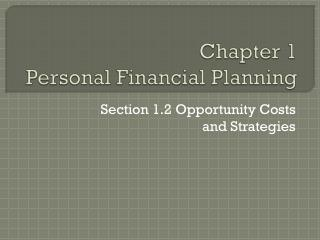DownloadDownload PresentationChapter 1 Personal Financial Planning

Chapter 1 Personal Financial Planning

Télécharger la présentationChapter 1 Personal Financial Planning

- - - - - - - - - - - - - - - - - - - - - - - - - - - E N D - - - - - - - - - - - - - - - - - - - - - - - - - - -
Presentation Transcript

1. Chapter 1Personal Financial Planning Section 1.2 Opportunity Costs and Strategies

2. Personal Opportunity Costs Personal resources require management just like financial resources. You have to make choices how you use your time. Would you rather complete your homework during free time at school or finish it when you get home?

3. Financial Opportunity Costs • You also have to make choices about how you use your money. • Would you buy the \$129 shoes or save money? Can’t do both. • Time Value of Money • The increase of an amount of money due to earned interest or dividends.

4. Calculating Interest • What you need to know: • Principal (PV) • original amount of money on deposit. • Annual Interest Rate (IY) • Time Frame or Number of Years (N)

5. Calculating Interest You just deposited \$1,000 in a savings account. The bank will pay you 3 percent annual interest. How much interest will you earn if you keep your money in the bank? Formula: Principal x Annual Interest Rate = Interest Earned Solution: \$1,000 x .03 = \$30

6. Calculating Interest - Practice You deposited \$50. Annual Interest 4%. Time 1 year. What are the earnings? Formula: Principal x Interest = Earnings Solution: \$50 x .04 = \$2

7. Calculating Future Value What you need to know: Future value is the amount your original deposit will be worth in the future based on earning a specific interest rate over a specific period of time. (\$1000 + \$30) x .03 = \$30.90 \$1030 + \$30.90 = \$1060.90

8. Calculating Future Value • You deposit \$2000. Annual Interest 5%. Time 3 years. What are the earnings? • What is the future value? • Easiest way? • Excel Spreadsheet

9. Achieving Your Financial Goals Obtain Plan Spend Wisely Save Borrow Wisely Invest Manage Risk Plant for Retirement